# Building a Financial Model

## This course explains how to set up the Excel spreadsheet and complete the initial steps of building a financial model, structuring calculations, and looking into costs and revenues, time periods, cash balances and reporting.

This course is not currently available

## This course will enable you to

• Set up and build a comprehensive financial model
• Calculate costs and revenues
• Decide which time periods you need to use and when and where to use flags
• Create understandable and useful reports

Every financial model is built on the same foundations: an understanding of its purpose, who it is for, and its scope. This course explains how to set up the Excel spreadsheet and complete the initial steps of building a financial model, structuring calculations, and looking into costs and revenues, time periods, cash balances and reporting.

## Look inside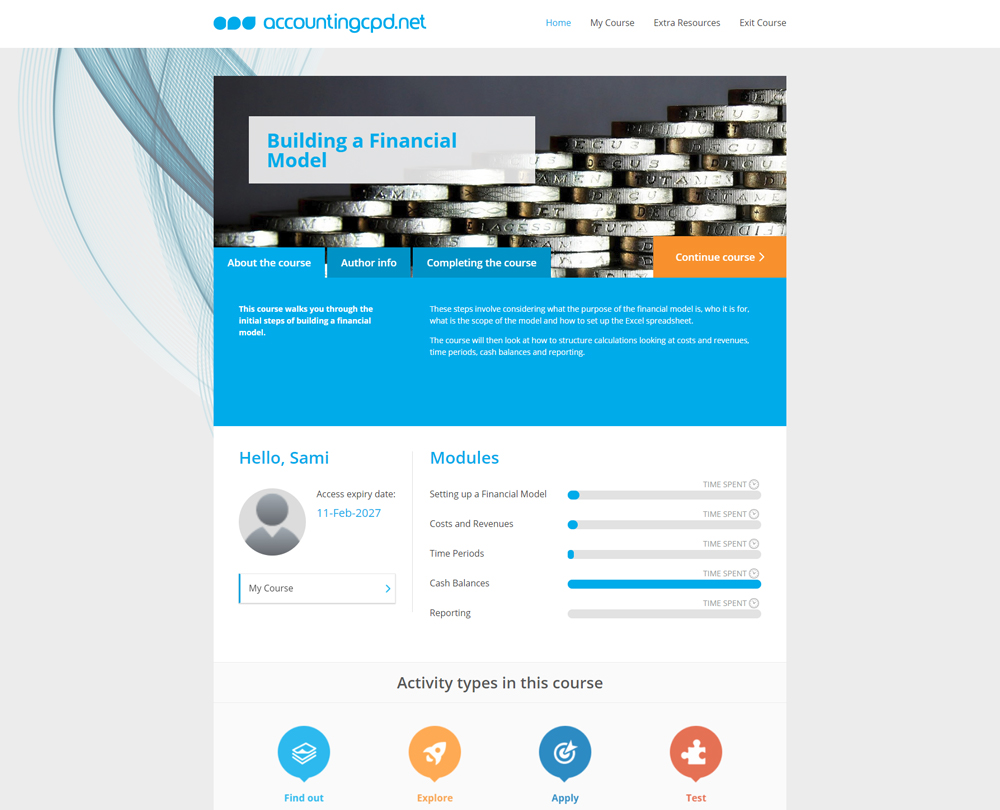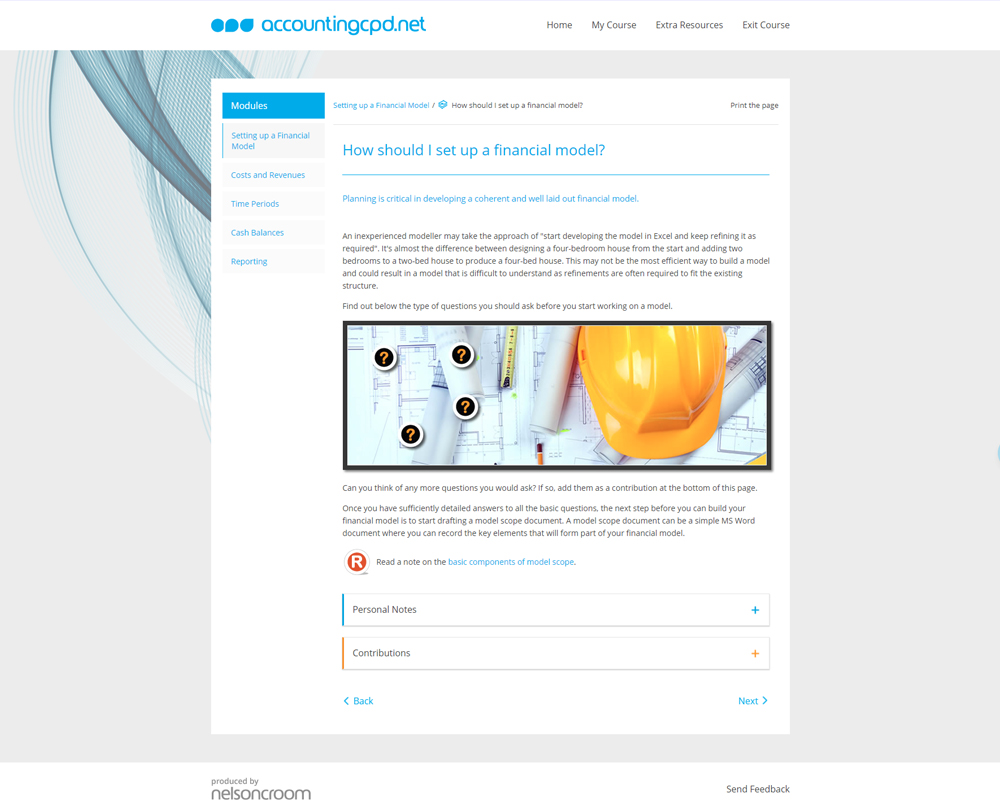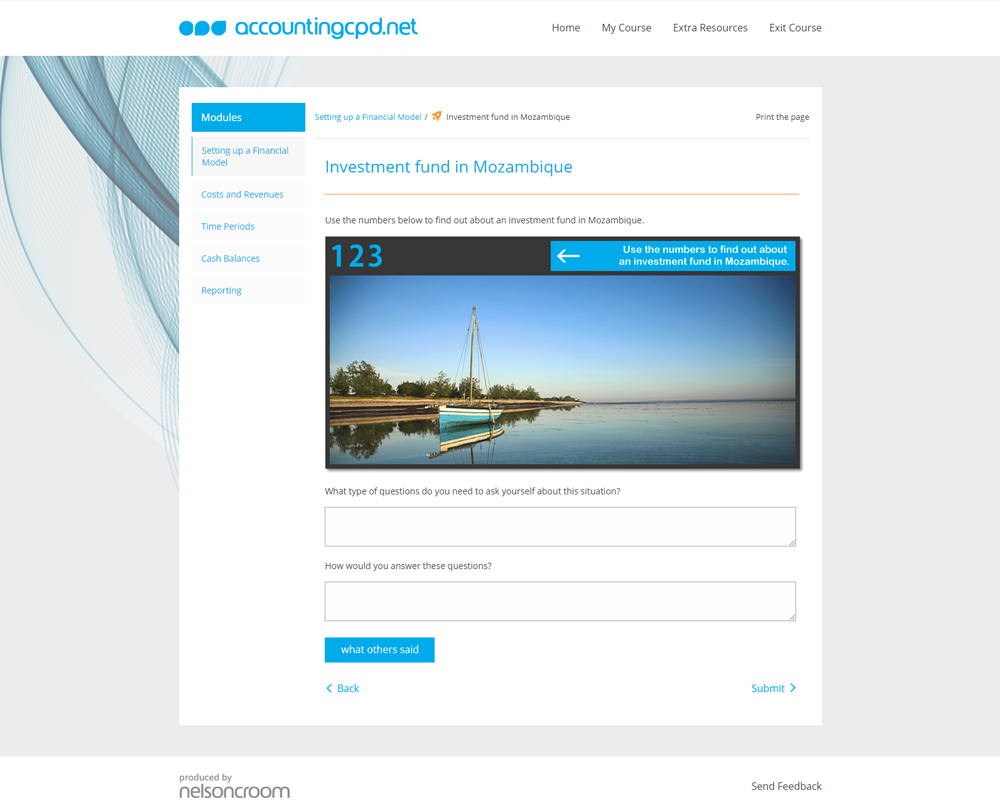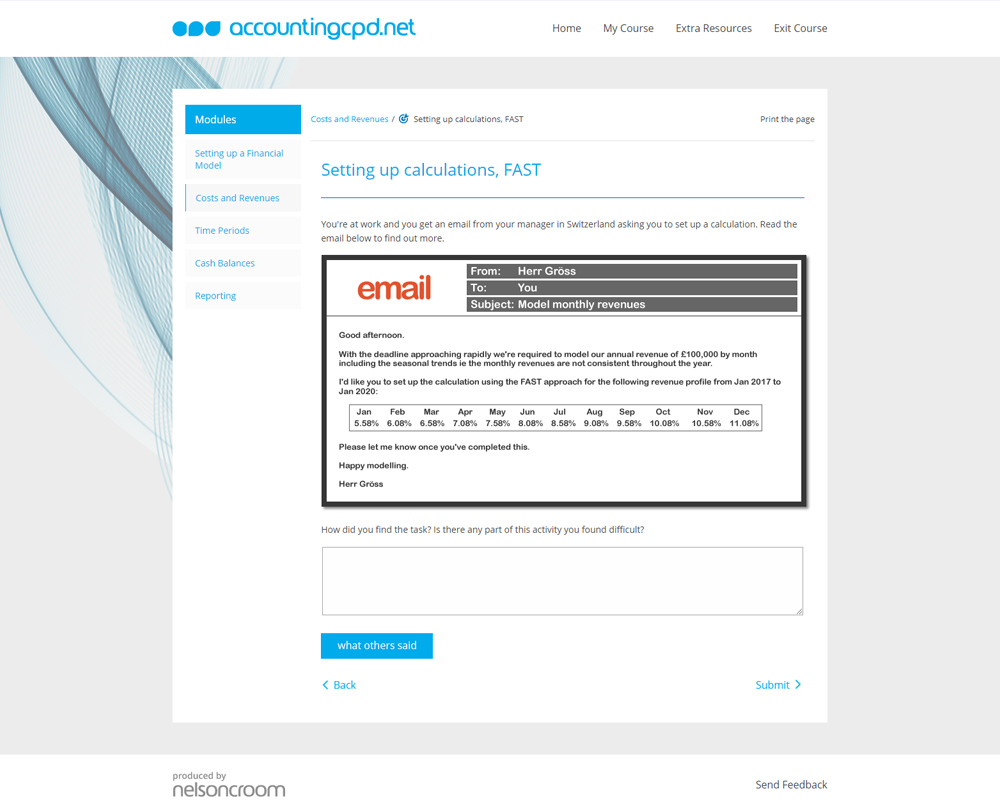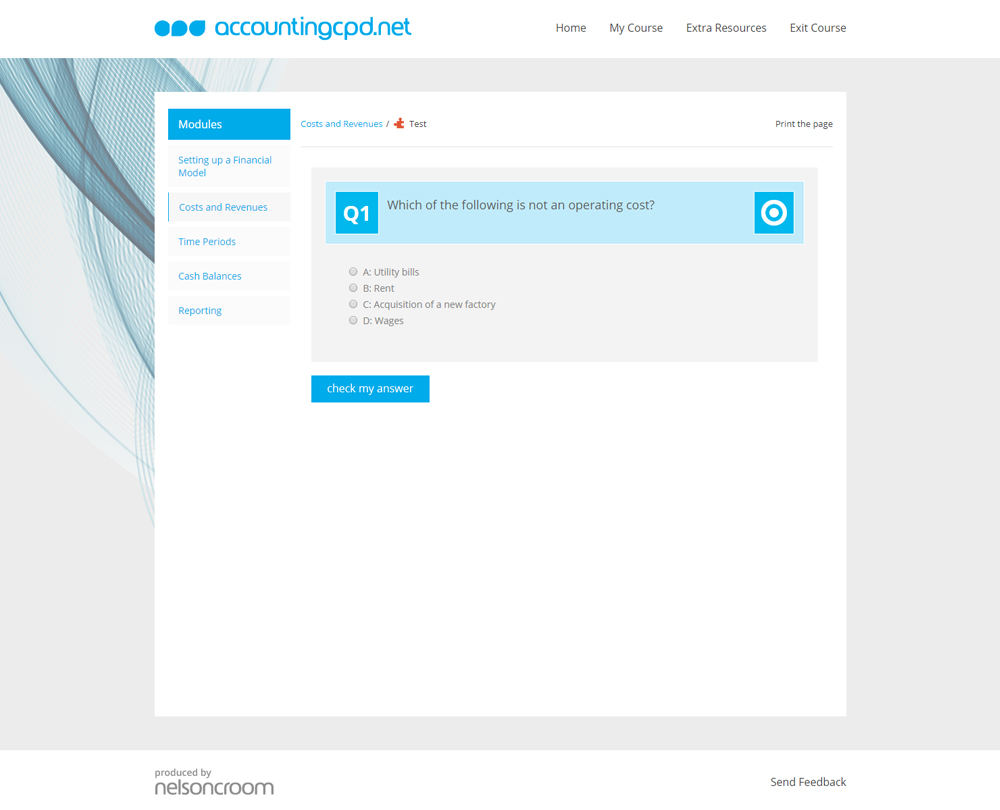## Contents

• Setting up a Financial Model
• Who uses a financial model?
• How should I set up a financial model?
• What are the MS Excel settings for financial model building?
• What should be included in a template?
• How should I incorporate FAST principles?
• Costs and Revenues
• What are the different ingredients required to calculate the operating costs?
• What would the concept diagram look like?
• How do I build a calculation that I can use to model the operating costs?
• What would be different in the revenue calculation?
• How do I build a calculation to quickly model the revenue?
• Time Periods
• Why is the concept of time important in modelling?
• What time periods should I use?
• What do I need to know about flags?
• Which ingredients are required to calculate the forecast period flag?
• Cash Balances
• Why is the cash balance particularly important?
• What is a "balance corkscrew"?
• What should I know about interest?
• How is interest calculated?
• Reporting
• What will the recipient want from the summary report?
• Should I use the same rules for building output sheets?
• How do I construct the output sheet?

## You might also like

Take a look at some of our bestselling courses

This course is not currently available. To find out more, please get in touch.